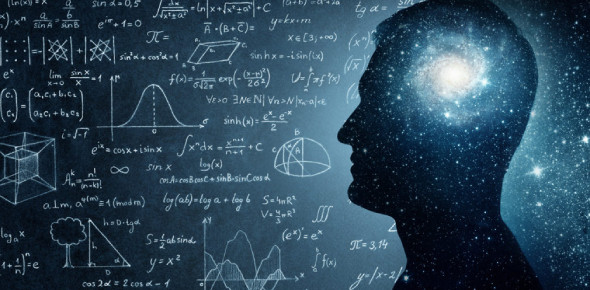# Trivia: Physics MCQ Quiz!

10 Questions | Total Attempts: 1978Settings.

• 1.
Frequency is measured in...
• A.

Ampere

• B.

Metres

• C.

Ohms

• D.

Hertz

• 2.
Sound cannot travel through...
• A.

Solid

• B.

Liquid

• C.

Vacuum

• D.

Gas

• 3.
What is force divided by the of the point of application?
• A.

Power

• B.

Pressure

• C.

Workdone

• D.

Energy

• 4.
Why does a sharp knife cut so well?
• A.

It has a large area so pressure is large

• B.

It has a large area so pressure is small

• C.

It has a small area so pressure is large

• D.

It has a small area so pressure is small

• 5.
What is normal in a ray diagram? It is the line drawn at angle _____ to the mirror surface.
• A.

180"

• B.

60"

• C.

40"

• D.

90"

• 6.
Which of the following is the correct reason why we can see non-luminous objects?
• A.

They bend light into our eyes

• B.

Our light gives out light

• C.

They shine light into our eyes

• D.

They reflect light into our eyes

• 7.
Which of the following is not true about light? Light is...
• A.

Fastest in vacuum

• B.

Slower than sound

• C.

Fastest in water

• D.

Fastest in Air

• 8.
What is the difference between weight and mass?
• A.

Mass is the quantity of substance while weight is a force

• B.

Mass is a force while weight is the quantity of substance

• C.

They are synonymous

• D.

Mass is heavier than weight

• 9.
The loss or gain of an electron in an atom result in the formation of...
• A.

Molecules

• B.

Ions

• C.

Positive charge

• D.

Negative charge

• 10.
In an experiment, a stone and a feather are thrown from the same height. Neglecting air resistance, which will hit the ground first?
• A.

The stone

• B.

The feather

• C.

Both will hit the ground the same time

• D.

The one that is heavier

Related TopicsBack to top